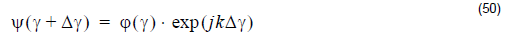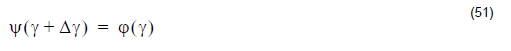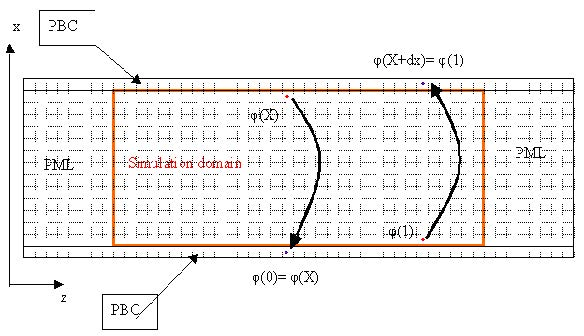This version of OptiFDTD provides the option to use simplified Periodic Boundary
Condition (PBC). PBC can work with other boundary conditions such as Anisotropic
PML, PMC, and PEC. With PBC, you can generate a simple plane wave simulation
or periodic layout simulations.

The simplified PBC is based on the Bloch’s Theorem:

• For the periodic layout, the wave function is written as the combination of a cell periodic component and a wave-like component:• The equation above needs to have a pre-defined value for the k-vector; which is not so feasible for an FDTD simulation, especially for Pulse input.

Therefore, we simplified the application by setting the k-vector in one specific
direction as zero, which then leads to the input wave being the axis-propagated wave,
and then the equation becomes:In an OptiFDTD application, Δin the simplified is the periodic length or one
dimensional length the edge of which is set to periodic boundary condition.

φ(Y) is the field component at the edge of the simulation domain.

Ψ(y + Δy) is the corresponding field value at the boundary of the opposite edge of
the φ(Y).

The following graph shows the relationship.